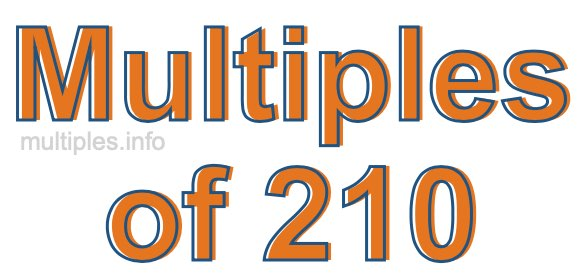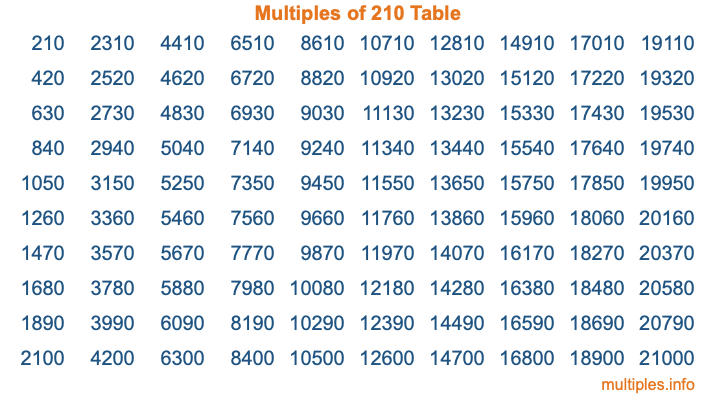Multiples of 210Welcome to the Multiples of 210 page. Here we will first teach you everything you will ever need to know about the multiples of 210, and then give you a study guide summary of everything we taught you to make sure you remember it all. Use this page to look up facts and learn information about the multiples of 210. This page will make you a multiples of two hundred ten expert!

Definition of Multiples of 210
Multiples of 210 are all the numbers that when divided by 210 equal an integer. Each of the multiples of 210 are called a multiple. A multiple of 210 is created by multiplying 210 by an integer.

Therefore, to create a list of multiples of 210, you start with 1 multiplied by 210, then 2 multiplied by 210, then 3 multiplied by 210, and so on for as long as you want. Thus, the list of the first five multiples of 210 is 210, 420, 630, 840, and 1050. To see a larger list of multiples of 210, see the printable image of Multiples of 210 further down on this page. We also have a category where you can choose any nth multiple of 210.

Multiples of 210 Checker
The Multiples of 210 Checker below checks to see if any number of your choice is a multiple of 210. In other words, it checks to see if there is any number (integer) that when multiplied by 210 will equal your number. To do that, we divide your number by 210. If the the quotient is an integer, then your number is a multiple of 210.

Is  a multiple of 210?

Least Common Multiple of 210 and ...
A Least Common Multiple (LCM) is the lowest multiple that two or more numbers have in common. This is also called the smallest common multiple or lowest common multiple and is useful to know when you are adding our subtracting fractions. Enter one or more numbers below (210 is already entered) to find the LCM.

Check out our LCM Calculator if you need more details about the Least Common Multiple or if you need the LCM for different numbers for adding and subtraction fractions.

nth Multiple of 210
As we stated above, 210 is the first multiple of 210, 420 is the second multiple of 210, 630 is the third multiple of 210, and so on. Enter a number below to find the nth multiple of 210.

th multiple of 210

Multiples of 210 vs Factors of 210
210 is a multiple of 210 and a factor of 210, but that is where the similarities end. All postive multiples of 210 are 210 or greater than 210. All positive factors of 210 are 210 or less than 210.

Below is the beginning list of multiples of 210 and the factors of 210 so you can compare:

Multiples of 210: 210, 420, 630, 840, 1050, etc.

Factors of 210: 1, 2, 3, 5, 6, 7, 10, 14, 15, 21, 30, 35, 42, 70, 105, 210

As you can see, the multiples of 210 are all the numbers that you can divide by 210 to get a whole number. The factors of 210, on the other hand, are all the whole numbers that you can multiply by another whole number to get 210.

It's also interesting to note that if a number (x) is a factor of 210, then 210 will also be a multiple of that number (x).

Multiples of 210 vs Divisors of 210
The divisors of 210 are all the integers that 210 can be divided by evenly. Below is a list of the divisors of 210.

Divisors of 210: 1, 2, 3, 5, 6, 7, 10, 14, 15, 21, 30, 35, 42, 70, 105, 210

The interesting thing to note here is that if you take any multiple of 210 and divide it by a divisor of 210, you will see that the quotient is an integer.

Multiples of 210 Table
Below is an image of the first 100 multiples of 210 in a table. The table is in chronological order, column by column. The first column has the first ten multiples of 210, the second column has the next ten multiples of 210, and so on.The Multiples of 210 Table is also referred to as the 210 Times Table or Times Table of 210. You are welcome to print out our table for your studies.

Negative Multiples of 210
Although not often discussed or needed in math, it is worth mentioning that you can make a list of negative multiples of 210 by multiplying 210 by -1, then by -2, then by -3, and so on, to get the following list of negative multiples of 210:

-210, -420, -630, -840, -1050, etc.

Multiples of 210 Summary
Below is a summary of important Multiples of 210 facts that we have discussed on this page. To retain the knowledge on this page, we recommend that you read through the summary and explain to yourself or a study partner why they hold true.

There are an infinite number of multiples of 210.

A multiple of 210 divided by 210 will equal a whole number.

210 divided by a factor of 210 equals a divisor of 210.

The nth multiple of 210 is n times 210.

The largest factor of 210 is equal to the first positive multiple of 210.

210 is a multiple of every factor of 210.

210 is a multiple of 210.

A multiple of 210 divided by a divisor of 210 equals an integer.

210 divided by a divisor of 210 equals a factor of 210.

Any integer times 210 will equal a multiple of 210.

Multiples of a Number
Here you can get the multiples of another number, all with the same attention to detail as we did for multiples of 210 on this page.

Multiples of
Multiples of 211
Did you find our page about multiples of two hundred ten educational? Do you want more knowledge? Check out the multiples of the next number on our list!